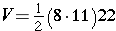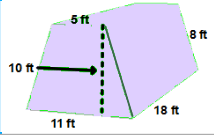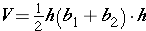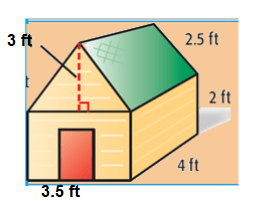# Volume

Last year, you learned to find the volumes of rectangular prisms. In this lesson, you will use the math you already know to solve problems involving volume of composed figures. Remember that the formula for finding volume is the same for all shapes:

V = Bh (where B is the area of the base and h is the height of the shape)

When you find the area of composite figures, you add the areas to get the area of the total shape. When you find the volume of composed figures, you add the volumes of each shape to get the total volume. Volume is always measured in cubic units.Use the formula V = Bh and insert the dimensions.

V = (7×7)12

V=(49)12

V=588Try it with a triangular prism:Use the formula V = Bh and insert the dimensions.(area of the triangle times the height of the prism)V = 44*22

V=968Time for a trapezoidal prism:Use the formula V = Bh and insert the dimensions.(area of the trapezoid times the height of the prism)V = 80 * 18It is time to put our knowledge of calculating volume into solving a problem with composed shapes. Many buildings are composed of a rectangular prism and a triangular prism. Solve the problem below:Mr. Stringer is building a garage for his toddler’s toy car. What is the volume of the garage?

Roof (triangular prism)
bh = 3.5 x 3 = 10.5
1/2 bh = 10.5/2 = 5.25 = B

Bh = 5.25 x 4 = 21 cubic ft

Base (rectangular prism)
lw = 3.5 x 4 = 14
lwh = 14 x 2 = 28
28 cubic ft

Roof + Base = 21 ft³ + 28 ft³ = 49 ft³

The garage has a volume of 49 cubic feet

## Volume Practice

A cube has six sides, or faces. Each of the six faces is a square.This cube measures 2 units on each edge. The faces of the cube are painted. Questions 1-4 are about this 2x2x2 cube.
1.  How many small cubes make up the large cube?
2. How many small cubes are painted on 3 faces?3. How many small cubes are painted on 2 faces?
4. How many small cubes are painted on 1 face?
———————————————————————————–5. How many small cubes make up the large cube?
6. If the large cube is painted, how many small cubes will be painted on 3 faces?
7. How many small cubes will be painted on 2 faces?
8. How many small cubes will be painted on 1 face?
———————————————————————————–10. If the large cube is painted, how many small cubes will be painted on 3 faces?